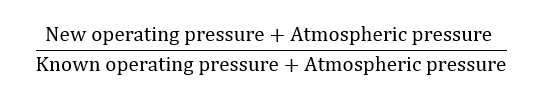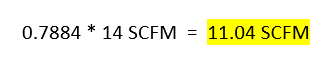## Absolute Pressure RatioThis model 1101 Super Air Nozzle requires 14 SCFM @ 80 PSIG. How much air will it consume at 60 PSIG?

Compressed air driven devices are always given a specification for the compressed air flow at a certain pressure.  For example, an EXAIR model 1101 Super Air Nozzle has a specified flow of 14 SCFM at 80 PSIG.  This means that when this nozzle is operated at 80 PSIG, it will require 14 SCFM of compressed air flow.  But what if the force from the nozzle is too high when operated at 80 PSIG and a lower operating pressure is needed?

Thankfully, we can calculate the compressed air flow at a different pressure using the absolute pressure ratio.  The absolute pressure ratio says that for any given change in absolute operating pressure, there will be a proportional change in the air consumption of a device.  So, what is an absolute pressure?

Put simply, an absolute pressure is the value which you would measure on pressure gauge plus the atmospheric pressure (PSIA, or Pounds per Square Inch Atmospheric).  So, our 80 PSIG operating pressure mentioned above is an absolute pressure of 94.5 PSI (80PSIG + 14.5 PSIA).  Similarly, if we wanted to determine the compressed air flow at an operating pressure of 60 PSIG, our absolute pressure would be 74.5 PSI (60 PSIG + 14.5 PSIA).The absolute pressure ratio is a ratio of the new absolute operating pressure (new PSIG + PSIA) compared to the known absolute operating pressure (known PSIG + PSIA).  For example, when comparing an operating pressure of 60 PSIG to an operating pressure of 80 PSIG, we will end up with the following ratio:This means that our absolute pressure ratio in this case is 0.7884.  To determine the compressed air flow for the model 1101 Super Air Nozzle at 60 PSIG, we will take this ratio value and multiply it by the known flow value at 80 PSIG.  This will yield the following:Utilizing this formula allows us to truly compare a compressed air powered device at different operating pressures.  If we did not use the absolute pressures when comparing compressed air devices at differing pressures, our values would be erroneously low, which could yield to improper compressed air system planning and performance.  And, using the absolute pressure ratio allows anyone to make a true comparison of compressed air device performance.  If specifications are given at different pressures, performance data can be misleading.  But, by using the absolute pressure ratio we can make a more exact evaluation of device operation.

If you have a question about your compressed air device and/or how a change in pressure will impact compressed air flow, contact our Application Engineers.  We’ll be happy to help.

Lee Evans
Application Engineer
LeeEvans@EXAIR.com
@EXAIR_LE